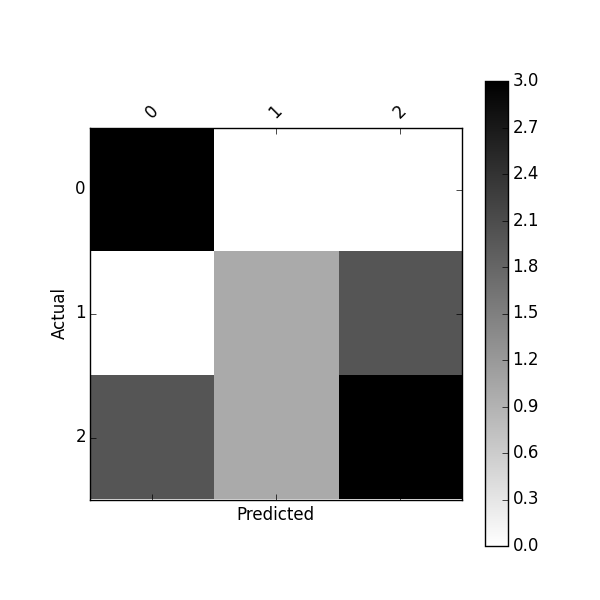2 views

I wrote a confusion matrix calculation code in Python:

def conf_mat(prob_arr, input_arr):

# confusion matrix

conf_arr = [[0, 0], [0, 0]]

for i in range(len(prob_arr)):

if int(input_arr[i]) == 1:

if float(prob_arr[i]) < 0.5:

conf_arr = conf_arr + 1

else:

conf_arr = conf_arr + 1

elif int(input_arr[i]) == 2:

if float(prob_arr[i]) >= 0.5:

conf_arr = conf_arr +1

else:

conf_arr = conf_arr +1

accuracy = float(conf_arr + conf_arr)/(len(input_arr))

prob_arr is an array that my classification code returned and a sample array is like this:

[1.0, 1.0, 1.0, 0.41592955657342651, 1.0, 0.0053405015805891975, 4.5321494433440449e-299, 1.0, 1.0, 1.0, 1.0, 1.0, 1.0, 0.70943426182688163, 1.0, 1.0, 1.0, 1.0]

input_arr is the original class labels for a dataset and it is like this:

[2, 1, 1, 1, 1, 1, 2, 1, 1, 2, 1, 1, 2, 1, 2, 1, 1, 1]

What my code is trying to do is: I get prob_arr and input_arr and for each class (1 and 2) I check if they are misclassified or not.

But my code only works for two classes. If I run this code for multiple classed data, it doesn't work. How can I make this for multiple classes?

For example, for a data set with three classes, it should return: [[21,7,3],[3,38,6],[5,4,19]]

by (33.1k points)

Scikit-Learn provides a confusion_matrix function

from sklearn.metrics import confusion_matrix

y_actu = [2, 0, 2, 2, 0, 1, 1, 2, 2, 0, 1, 2]

y_pred = [0, 0, 2, 1, 0, 2, 1, 0, 2, 0, 2, 2]

confusion_matrix(y_actu, y_pred)

which output a Numpy array

array([[3, 0, 0],

[0, 1, 2],

[2, 1, 3]])It is the most common method to get a confusion matrix in python.